Courses
Courses for Kids
Free study material
Free LIVE classes
More

# LR Circuit and RC Circuit - JEE## LR and RC Circuit: An Introduction

Last updated date: 28th Mar 2023
Total views: 67.2k
Views today: 0.43k

We all are aware of the terms inductor, capacitor, and resistor. An inductor is a two-terminal passive component which stores energy in the form of magnetic field when electric current flows through it. It is in the shape of a coil made out of an insulated wire. Resistor is a two-terminal passive component which stores electric charge when electric current passes through it. A resistor is also a two-terminal passive component which applies resistance in any circuit. There are different combinations made out of these three components for different applications in circuits such as LCR circuit, RC circuit, and LR circuit. They are mostly used as filters.

In this article, we are going to understand LR circuits and RC circuits with proper circuit diagrams. Also, we calculate the time constant and the steady state current of the LR and RC circuit.

## What is an LR Circuit?

An inductor-resistor circuit (LR circuit) is a combination of resistors and inductors. These two components, i.e., resistor and inductor can be connected into series or parallel. Thus, LR circuits are of two types – series LR circuit and parallel LR circuit.

In a series LR circuit, the driving source of the circuit is voltage, whereas in a parallel LR circuit, the driving source of the circuit is current. They are commonly used as passive filters. The circuit diagram given below is a first-order LR circuit with one inductor and resistor. Let’s understand the circuit and calculate the time constant and the steady state current of LR circuits.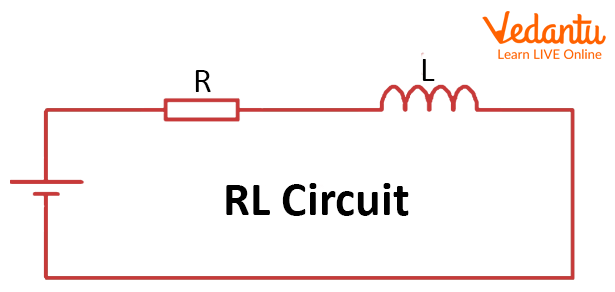LR Circuit

## LR Circuit Diagram

In order to understand the LR circuit, let’s take a first-order series LR circuit which consists of one inductor and one capacitor.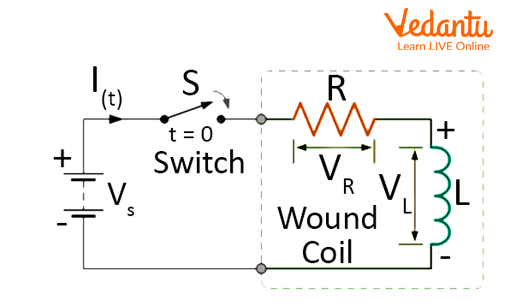Series LR Circuit

To calculate the current through the circuit and time constant, we can use Kirchhoff’s voltage law, which states that the sum of the voltage due to the resistance as well as the inductance should be zero.

So, $V\left( t \right)\text{ }=\text{ }{{V}_{R}}~+\text{ }{{V}_{L}}$

The voltage in resistor is ${{V}_{R}}~=\text{ }I{{R}_{~}}$

And, the voltage in inductor is $VL=\frac{Ldi}{dt}$

The total voltage V(t) is

$V\left( t \right)=IR+\text{ }\frac{Ldi}{dt}$

The total current is

$~I(t)={{I}^{0}}[1-{{e}^{-t}}^{\tau }]$

$~I(t)=\frac{V}{R}[1-{{e}^{-\frac{Rt}{L}}}]$

Thus, time constant $\tau =LR$ and

Steady-state current $I=VR$

Also, the transient curve for an LR series circuit is depicted below: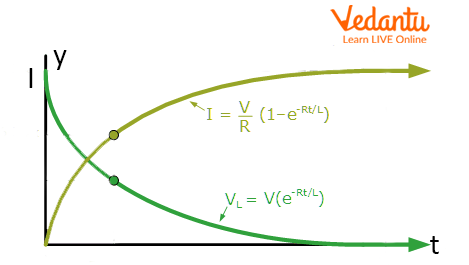Time vs Current Graph

## Series RL Circuit

The circuit diagram of a series RL circuit is given below: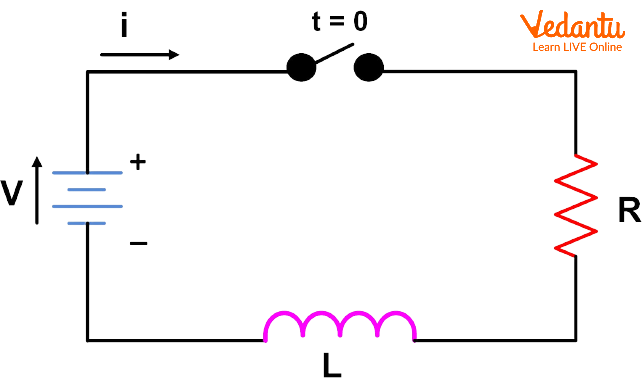Series RL Circuit

## What is an RC Circuit?

A resistor-capacitor circuit (RC circuit) is a combination of resistors and capacitors. These two components, i.e., resistor and capacitor can be connected into series or parallel. Thus, RC circuits are of two types – series RC circuit and parallel RC circuit.

In a series RC circuit, the driving source of the circuit is voltage, whereas in a parallel RC circuit, the driving source of the circuit is current. They are used to create crude filters like low-pass, high-pass, and band-pass. The circuit diagram given below is a first-order RC circuit with one resistor and capacitor. Let’s understand the circuit and calculate the time constant and the steady state current of RC circuits.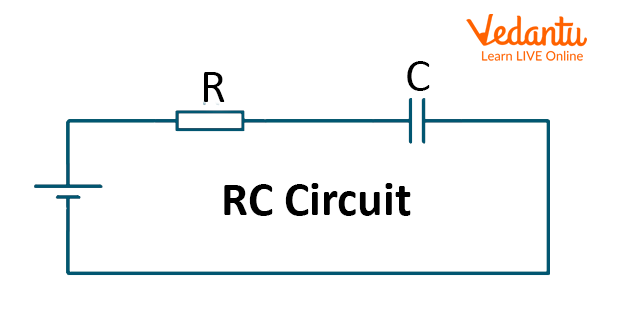RC Circuit

## RC Circuit Diagram

In order to understand the RC circuit, let’s take a first-order series RC circuit which consists of one resistor and one capacitor.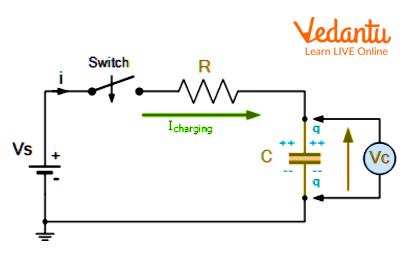RC Series Circuit

Again on applying Kirchoff’s voltage law,

$\frac{q}{c}=IR$

As $I=\frac{dq}{dt}$

So, $qc=\frac{dq}{dtR}$

As we know,

$\Rightarrow q={{q}_{0}}{{e}^{\frac{-t}{t}}}$

$\Rightarrow I=\frac{dq}{dt}$

On substituting,

$\frac{dq}{dt}=\frac{d({{q}_{0}}{{e}^{\frac{-t}{T}}})}{dt}$

$\Rightarrow I={{I}_{0}}{{e}^{\frac{-t}{t}}}$

$\Rightarrow I=\frac{V}{R}{{e}^{\frac{-t}{T}}}$

Thus, time constant $t=RC$ and

Steady state current ${{I}_{0}}=\frac{V}{R}$

## Current in RC Circuit

The current in RC circuit is calculated by the formula

${{I}_{0}}=\frac{V}{R}$

## Summary

An inductor-resistor circuit (LR circuit) is a combination of resistors and inductors, whereas a resistor-capacitor circuit (RC circuit) is a combination of resistors and capacitors. In both LR and RC circuits, the two components can be connected in either series or parallel. Depending upon the type of connections, LR and RC circuits are divided into two types, i.e., series LR circuit, parallel LR circuit, and series RC circuit, parallel RC circuits. In series, the driving source of the circuit is voltage, whereas in parallel, the driving source of the circuit is current. They are commonly used as filters.

By using Kirchoff’s voltage law, and solving by substituting the values, the time constant for LR circuit is $\tau =LR$ and the steady state current is $I=VR$, whereas the time constant of RC circuit is $t=RC$ and the steady state current is ${{I}_{0}}=\frac{V}{R}$.

## FAQs on LR Circuit and RC Circuit - JEE

1. Where are RC circuits used?

In a circuit, capacitors and resistors are frequently seen together. These RC circuits are used often in daily life. They regulate many different electronic equipment, including heart pacemakers, camera flashes, the speed of a car's windshield wipers, and the timing of traffic signals.

2. Why is the resistor used in RC circuits?

The resistor, abbreviated as "R," is one of the crucial components of an RC circuit. A resistor passively blocks the flow of current in an electrical circuit. It 'resists,' the flow of electrons through the circuit.